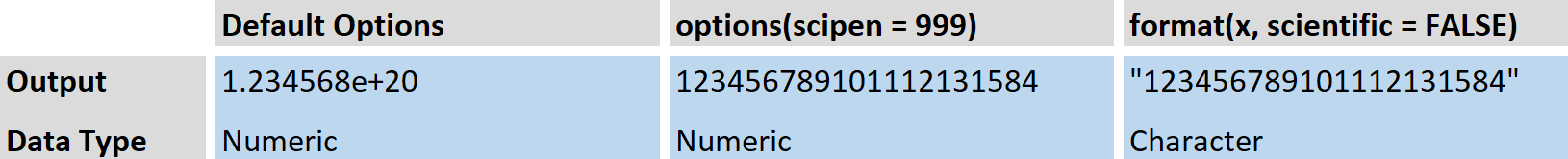# Disable Scientific Notation in R (2 Examples) | How to Prevent Exponential Numbers

In this tutorial, you will learn how to disable scientific notation (e.g. e+10) in the R programming language. The article will be structured as follows:

Figure 1 shows a quick overview of the following examples:Figure 1: Overview of Number Representations in R.

However, for further explanations keep on reading…

## Create Example Data

In the examples of this article, I will use the following numeric data object:

```x &lt;- 123456789101112131415 # Example data object x # Print example data to console # 1.234568e+20```

We will use the number 123456789101112131415, which is stored in the data object x.

As you can see based on the previous RStudio console output, base R formats this number automatically to an exponential notation (i.e. 1.234568e+20).

In the following examples, I will show you two ways how to disable this notation in R.

So without further ado, let’s move on to the examples…

## Example 1: Modify R Options to Disable Scientific Notation

A general approach is to change the options within R. You just need to execute the following syntax in order to tell R that it should not show scientific notation anymore:

`options(scipen = 999) # Modify global options in R`

Let’s see what happens when we print our example data to the RStudio console again:

```x # Print example data once more # 123456789101112131415```

The exponential representation was removed and all digits are shown.

Keep in mind that R does not store very large numbers “precisely”, so for that reason, the numbers shown in the output differ from our example data object. You can find more on this here.

Note: With the previous code, we changed the general R settings. If you want to move back to the old settings you can either restart RStudio; Or you can back up the default options before changing them; Or…

…you might use the syntax shown in the next example, where we switch off scientific notation for only one specific number!

## Example 2: Disable Scientific Notation with the format R Function

The R format function enables us to prevent R from showing an exponential representation. Have a look at the following R code:

```format(x, scientific = FALSE) # Apply format function in R # "123456789101112131584"```

As you can see, the whole number with all digits was returned to the RStudio console.

Note: This number was converted to the character class. If you want to perform calculations with the converted output, you need to transform this character back to numeric.

## Further Resources for Dealing with Exponential Notation

Handling scientific notation can be confusing. If you want to learn more about scientific notation in general, I can recommend the following YouTube video of Tyler DeWitt. In the video he explains how scientific notation works in general:

Please accept YouTube cookies to play this video. By accepting you will be accessing content from YouTube, a service provided by an external third party.If you accept this notice, your choice will be saved and the page will refresh.

Furthermore, you might have a look at the other articles of this website. I’m publishing R tutorials on the handling of numeric data regularly:

In this tutorial, I have shown you how to get rid of exponential notation in R. However, if you have further questions on this or any other statistical topic, don’t hesitate to let me know in the comments below!

Subscribe to the Statistics Globe Newsletter